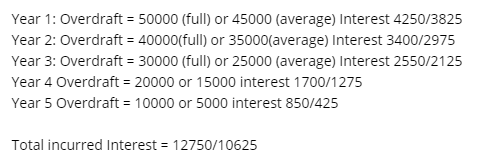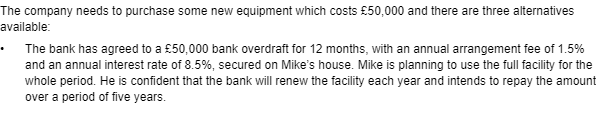Overdraft calculation (AAT L4 mock exam Q8)

Hi, could anyone explain the 2 ways of calculating the overdraft interest in this question? I’ve looked at the answer and still can’t get my head around how they worked it out!

• So for the overdraft option regardless of the method picked you need to calculate the total cost.

The £50,000 is the loan amount and the arrangement fee is 5*50000*0.015 =£3,750.

Interest for each year can be done on either a start of year balance or an average for the year.

Year 1: Overdraft = 50000 (full) or 45000 (average) Interest 4250/3825
Year 2: Overdraft = 40000(full) or 35000(average) Interest 3400/2975
Year 3: Overdraft = 30000 (full) or 25000 (average) Interest 2550/2125
Year 4 Overdraft = 20000 or 15000 interest 1700/1275
Year 5 Overdraft = 10000 or 5000 interest 850/425

Total incurred Interest = 12750/10625

You then add the arrangement fee for the total interest paid hope this helps explain how this works.
AAT Level 4, MAAT
ACCA in progress
F4- Passed Aug 2020
F5- Passed Dec 2020
F6- Passed Sep 2020
F7- Passed June 2021
F8 - Passed Sep 2021
F9 - Passed June 2021

SBL
SBR
ATX - Planned Dec 21
APM -
• Amazing thank you so much really appreciate it!
• Hi,
Could you please help. When calculating cost of sales, when asked that 18% is gross operating profit. Does it minus in the gross profit amount before calculating cost of sales?
I really need to understand this one. Thank you
• Hi lovelyanna your question seems to be a bit muddled do you have the question it relates to?

Gross Profit = Sales - Cost of sales

Gross Profit Margin = (Sales - Cost of Sales)/Sales *100

I'm guessing you have a sales figure and a Gross Profit margin

CoS = Sales - 0.18 Sales would be your answer in that case.
AAT Level 4, MAAT
ACCA in progress
F4- Passed Aug 2020
F5- Passed Dec 2020
F6- Passed Sep 2020
F7- Passed June 2021
F8 - Passed Sep 2021
F9 - Passed June 2021

SBL
SBR
ATX - Planned Dec 21
APM -
• Hi Pian32, thank you for checking this one. No, I don't have because I could only remember this question from my last exam that I got stuck and don't know how to answer.

*CONTENT REMOVED AS RELATES TO LIVE ASSESSMENT*

The following should be calculated:
Revenue = ( COS + Gross Profit)
COS = (Revenue - Gross Profit)
Inv = (COS x Inv days/365)

I made a guessed on the days for Inv days = 34 days, trade receivable days 26 days = 60 days - trade payable days 19 = working capital cycle = 41 days, the days that I had provided will equate to 41 days.

I did it based on the video I watched in YouTube. It was stated that you need to sell your inventory quickly and less the payable days so that your cashflow will be much better.

I am stuck understanding this question because, I could not find a similar question in the workbook I used for my revision. Also, I bought a workbook from Osbourne but still waiting for the delivery. Is there anyway you can advise on how I can solve this one?. I would appreciate a lot if you can help me as I don't have any access to my previous tutor. Unless I will sign up again and re-do the whole tuition. The exam was my 3rd attempt. I am wondering if there is tutor that can help, like pay as you go arrangement, just asking...please help....
• So in this type of question you need to keep in mind all the ratios you have access to. In this case you don't want to use the revenue equation you have listed as there isn't enough information instead you'd use.

I'm still not sure what you mean by gross operating profit of 18% are you meaning a margin and if so which margin Gross or operating. (I didn't do this module so some of the terms/equations used in this module I might not be familiar with.)

If it is a margin then

Margin = Profit (margin appropiate) / Revenue *100

Revenue = Profit / Margin (as a decimal)

Are you calculating inventory or inventory days?

If the former then you'd need to be given the days or enough information in the statements if you have the latter

Inventory days = (Average Inv/COS) *365

You seemed fine with the other two.

If you have access to the financial statements and decision and control modules they both have a ratios section which might have some relevant questions that would help with this type of question.

These questions as a whole are understanding what can be calculated from each ratio. There are multiple ways to get the revenue figure for example from different ratios. You have the basic profit equation then the 2 ratios GPM and OPM as well as asset turnover. Similarly for the operating profit you can use the OPM ratio or the ROCE etc. Often you'll need to use one ratio to get the needed figure for another ratio.

AAT Level 4, MAAT
ACCA in progress
F4- Passed Aug 2020
F5- Passed Dec 2020
F6- Passed Sep 2020
F7- Passed June 2021
F8 - Passed Sep 2021
F9 - Passed June 2021

SBL
SBR
ATX - Planned Dec 21
APM -
• Hi @lovelyanna

I have had to remove some of your comment in the above post as it relates to Live assessments. Please note you are not permitted to discuss or disclose any information from live assessment materials. Please discuss these offline with your tutor or stick to practice assessment materials within the community.

Thanks
• Can someone please tell me what is mark-up and margin is- in simple terms. I hear that some students find it easy where as, i do not!
• Hi @Happy2021

I can try!

Mark-up and Margin are two ways of calculating profit.

Start with the basic relationship: cost of goods sold + profit = sales revenue

• Mark-up is a percentage of the cost of goods sold.

If your mark-up is 20%, then you take the cogs, find 20% of that (which is your profit), and the two added together give your revenue from sales.

e.g Say your cogs is £500. Then your profit is 20% of this (£500 * 0.2 = £100). Your revenue from sales will be the cogs + profit: the equation above becomes £500 + £100 = £600.
• Margin is a percentage of the revenue from sales.

If your margin is 20%, then take the revenue figure, calculate 20% (this is your profit), and the revenue minus the profit will be your cogs value.

e.g Say your revenue from sales is £500. Then your profit is 20% of this (£100 as calculated above). Your cogs was then £500 - £100 = £400. Then the equation above becomes £400 + £100 = £500.

I think of mark-up as starting with the lower figure (cogs) and literally increasing it with my mark-up (profit), to find my sales.

Whereas with margin I already have the larger sales figure - like a whole sheet of paper - and I have to find the lower amounts of cogs and profit hidden within it (in the way a sheet of paper can be divided between the margin and the area you'd actually write in).

A step onwards: if you know the mark-up but you know sales instead of cogs, you'll have to do a reverse % calculation. Similarly, if you know the margin but cogs instead of sales, you'll need to think an extra step to get to the figure you need to find.

Hope this helps a bit.
• Hi,

I'm going back to the original question on this thread of calculating the overdraft interest on AAT Level 4 Mock Assessment 1 Q8 (ii).
I've calculated the interest based on the start of year balance being £12,750, but cannot work out how the average overdraft of £10,625 is calculated. I've been going round in circles with this one!

In the example above the average balances are calculated: (screenshot from above response)I don't understand where the average overdraft balances are coming from. I've included a screenshot of the question for context.Can anybody explain how the average overdraft balances are calculated please?
• Average overdraft = (Overdraft at start of the year + Overdraft at end of year)/2

It treats it as if the overdraft was paid steadily over the year rather than in a £10,000 lump.

You then apply the interest rate to the average and sum them to get the total interest.
AAT Level 4, MAAT
ACCA in progress
F4- Passed Aug 2020
F5- Passed Dec 2020
F6- Passed Sep 2020
F7- Passed June 2021
F8 - Passed Sep 2021
F9 - Passed June 2021

SBL
SBR
ATX - Planned Dec 21
APM -
• @Pian32 I've got it now! Thank you for your help.